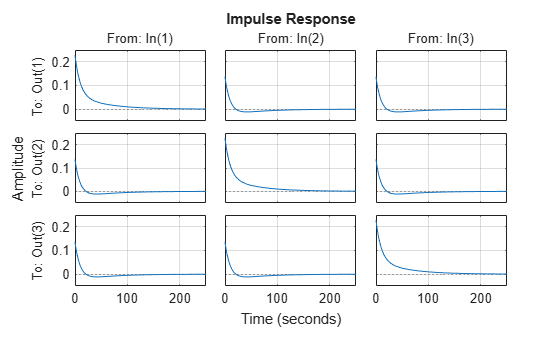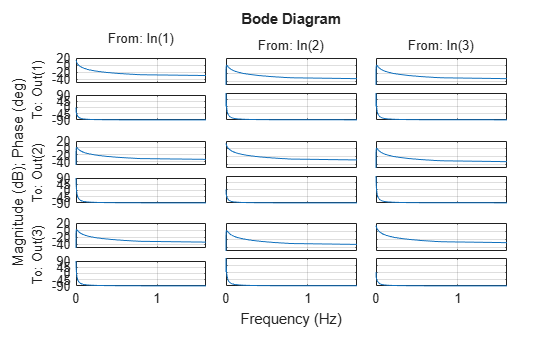# getoptions

Return plot options handle or plot options property

## Syntax

``p = getoptions(h)``
``p = getoptions(h,propertyName)``

## Description

You can use `getoptions` to obtain the plot handle options or properties list and use it to customize the plot, such as modify the axes labels, limits and units. For a list of the properties and values available for each plot type, see Properties and Values Reference (Control System Toolbox). To customize an existing plot using the plot handle:

1. Obtain the plot handle

2. Use `getoptions` to obtain the option set

3. Update the plot using `setoptions` to modify the required options

For more information, see Customizing Response Plots from the Command Line (Control System Toolbox).

example

````p = getoptions(h)` returns the plot options handle associated with plot handle `h`. `p` contains all the settable options for a given response plot.```

example

````p = getoptions(h,propertyName)` returns the specified options property, `propertyName`, for the plot with handle `h`. You can use this to interrogate a plot handle.```

## Examples

collapse all

For this example, consider a MIMO state-space model with 3 inputs, 3 outputs and 3 states. Create a impulse plot with red colored grid lines.

Create the MIMO state-space model `sys_mimo`.

```J = [8 -3 -3; -3 8 -3; -3 -3 8]; F = 0.2*eye(3); A = -J\F; B = inv(J); C = eye(3); D = 0; sys_mimo = ss(A,B,C,D); size(sys_mimo)```
```State-space model with 3 outputs, 3 inputs, and 3 states. ```

Create an impulse plot with plot handle `h` and use `getoptions` for a list of the options available.

`h = impulseplot(sys_mimo)````h = resppack.timeplot ```
`p = getoptions(h)`
```p = Normalize: 'off' SettleTimeThreshold: 0.0200 RiseTimeLimits: [0.1000 0.9000] TimeUnits: 'seconds' ConfidenceRegionNumberSD: 1 IOGrouping: 'none' InputLabels: [1x1 struct] OutputLabels: [1x1 struct] InputVisible: {3x1 cell} OutputVisible: {3x1 cell} Title: [1x1 struct] XLabel: [1x1 struct] YLabel: [1x1 struct] TickLabel: [1x1 struct] Grid: 'off' GridColor: [0.1500 0.1500 0.1500] XLim: {3x1 cell} YLim: {3x1 cell} XLimMode: {3x1 cell} YLimMode: {3x1 cell} ```

Use `setoptions` to update the plot with the required customization.

`setoptions(h,'Grid','on','GridColor',[1 0 0]);`The impulse plot automatically updates when you call `setoptions`. For MIMO models, `impulseplot` produces a grid of plots, each plot displaying the impulse response of one I/O pair.

For this example, consider a MIMO state-space model with 3 inputs, 3 outputs and 3 states. Create a Bode plot with linear frequency scale, specify frequency units in Hz and turn the grid on.

Create the MIMO state-space model `sys_mimo`.

```J = [8 -3 -3; -3 8 -3; -3 -3 8]; F = 0.2*eye(3); A = -J\F; B = inv(J); C = eye(3); D = 0; sys_mimo = ss(A,B,C,D); size(sys_mimo)```
```State-space model with 3 outputs, 3 inputs, and 3 states. ```

Create a Bode plot with plot handle `h` and use `getoptions` for a list of the options available.

```h = bodeplot(sys_mimo); p = getoptions(h)```
```p = FreqUnits: 'rad/s' FreqScale: 'log' MagUnits: 'dB' MagScale: 'linear' MagVisible: 'on' MagLowerLimMode: 'auto' PhaseUnits: 'deg' PhaseVisible: 'on' PhaseWrapping: 'off' PhaseMatching: 'off' PhaseMatchingFreq: 0 ConfidenceRegionNumberSD: 1 MagLowerLim: 0 PhaseMatchingValue: 0 PhaseWrappingBranch: -180 IOGrouping: 'none' InputLabels: [1x1 struct] OutputLabels: [1x1 struct] InputVisible: {3x1 cell} OutputVisible: {3x1 cell} Title: [1x1 struct] XLabel: [1x1 struct] YLabel: [1x1 struct] TickLabel: [1x1 struct] Grid: 'off' GridColor: [0.1500 0.1500 0.1500] XLim: {3x1 cell} YLim: {6x1 cell} XLimMode: {3x1 cell} YLimMode: {6x1 cell} ```

Use `setoptions` to update the plot with the requires customization.

`setoptions(h,'FreqScale','linear','FreqUnits','Hz','Grid','on');`The Bode plot automatically updates when you call `setoptions`. For MIMO models, `bodeplot` produces an array of Bode plots, each plot displaying the frequency response of one I/O pair.

## Input Arguments

collapse all

Plot handle, specified as a plot handle object. For example, `h` is a `mpzplot` object for a pole-zero or I/O pole-zero plot.

Specific property name, specified as a string or character vector. For a list of the properties and values available for each plot type, see Properties and Values Reference (Control System Toolbox).

## Output Arguments

collapse all

Plot options handle, returned as a plot options handle object. For example, `p` is a `PZMapOptions` object for a pole-zero or I/O pole-zero plot.

## Version History

Introduced in R2012a